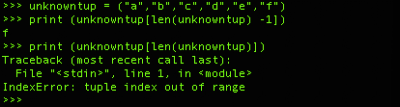Saturday, May 09, 2009

Python, using a tupleIn Python, as can been seen in some post I posted before this one, one can make use of tuples. A tuple is nothing more than a list. Wikipedia states the following on the subject of tuple:

In mathematics, a tuple is a sequence (or ordered list) of finite length. An n-tuple is a tuple with n elements. Tuples are usually written within parenthesis. For example, (2, 7, 4, 1, 7) is a 5-tuple. Tuples are often used to describe mathematical objects that consist of specified components. For example, a graph is commonly defined as the 2-tuple (V, E) where V is the set of vertices and E is the set of edges. The edge set E is a subset of the cartesian product V × V, hence a set of 2-tuples.

A tuple can widely be used within Python for all kinds of tasks, a first simple example is using a tuple to construct a sentence. The use of this example might not be clear at first for a day to day usage however it will illustrate the use of a tuple.In the above example we make use of a 2-tuple to construct the sentence. Now this example is not really useful in this setup because we could simply construct the entire sentence in one go and did not really have to make use of a tuple. However, in the below example you can see that if we define the content of a tuple first it already makes more sense.Now we understand that we can define a tuple outside the print function as shown in the first example it suddenly will make a lot more sense. Even do we could still construct the above statement by using 2 string variables in this case the use of a tuple will make things more easy.In the examples above we have used tuples to store strings, however in basic a tuple can hold any type of variable you need, so for example you can use it store also a int or a float. When you define a int or float inside a tuple it will also be in this variable type when you request it from the tuple. A example of this is below:All of this is working just fine as long as you know the length of the tuple, in some cases you will encounter a situation where you do not know the length of your tuple, aka you do not know how many "items" it contains. in this case the len() command comes in handy. You can do a len(x) where x is the name of your tuple. This will return the number of "items" in your tuple. Remember, when accessing a tuple we start with "item" 0 and NOT 1. so if you need to get the last "item" from the tuple it will be n -1 where n is the number of items inside the tuple. You can see this in the example below:In the last statement of the example above we do not a -1 on the len(x) in this case it is asking for unknowntup which is not inside you tuple and this will result in the indexerror tuple index out of range.